Fundamentals of Molecular Interaction Analyzer QCM

## QCM based on Admittance method (QCM-A).

In addition to the oscillation method often used in conventional QCM devices, applying a frequency to the crystal from the outside by using a network analyzer or impedance analyzer used for evaluation of electronic device components is another way to vibrate the crystal.
The frequency that is applied can be swept to obtain the characteristics near the crystal resonance point.
The AFFINIX QN Pro uses the latter method.

## QCM-A method：Principle of QCM based on Admittance method.

The characteristics (amplitude, phase, insertion loss, impedance, admittance) of a crystal resonator can be measured by sweeping frequencies near the resonant frequency of the crystal resonator using a network analyzer.
AFFINIX QN Pro mainly measures admittance and analyzes it by determining conductance (G): a real component of admittance, and susceptance (B): an imaginary component.
This method is called QCM-A method：QCM based on Admittance method.

The characteristics of the quartz crystal other than series resonance frequency Fs have been measured by analyzing the impedance and admittance of the quartz crystals. However, the measurement method of QCM that uses a quartz crystal as a sensor was not commonly used.This is because a high-frequency instrument for the measurement is expensive and the resolution of a frequency equivalent to that of the oscillation method cannot be obtained by the QCM measurement method, compared with an oscillation method which can get stable oscillation at low cost.
In order to solve the above problems, we studied measuring instruments and their measurement conditions, optimized the analysis algorithm of the obtained admittance, and succeeded in developing a measurement method with a frequency resolution close to that of the oscillation method.In addition to the measurement accuracy of the frequency which is almost equivalent to that of the oscillation method, the characteristics of the quartz crystal other than the resonance frequency can be obtained, which enables viscoelastic analysis and physical properties evaluation of adsorbates and solutions.

The figure below shows the frequency along the horizontal axis and the conductance, which is the real component of admittance, along the vertical axis, and shows the vicinity of the resonance frequency. The peak of the waveform indicates the resonance frequency.The difference of measurement between the admittance analysis method (QCM-A) and the oscillation circuit method is shown in the animation. The QCM-A method measures at "a point that is moving" in the measurement area, and the oscillation method measures at " a point that is fixed".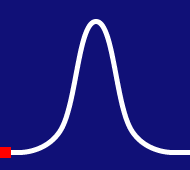■QCM based on Admittance method (QCM-A).
other than the electrical sweep frequency around the resonance frequency of the crystal unit can be acquired.
(Vertical axis: conductance, horizontal axis: frequency)■Oscillation method
The point that can oscillate stably without applying the load by oscillation circuit
＝Measure the oscillation point (resonance frequency). Only the resonance frequency is measured.
(Vertical axis: conductance, horizontal axis: frequency)

'Admittance' is the reciprocal of impedance and is divided into conductance G of the real part and susceptance B of the imaginary part.Impedance is a parameter that shows "resistance", the difficulty of the current flow of AC voltage. When the current becomes difficult to flow (high resistance), the impedance value increases, and the admittance value decreases.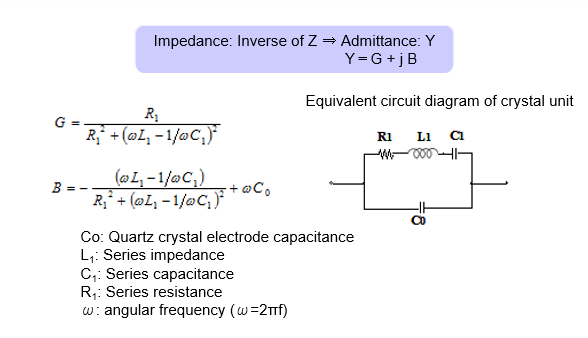The values of conductance G and susceptance B obtained from a measurement (one sweep) are illustrated, and a circle is drawn as shown below. This is called the admittance circle diagram.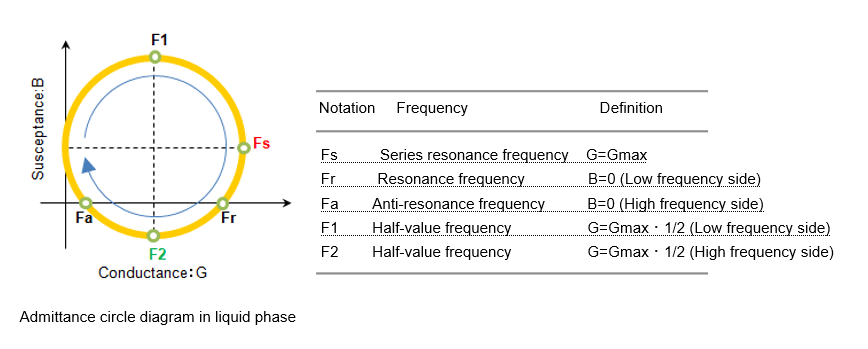There are frequencies with each characteristic on this circle diagram, but
AFFINIX QN Profinds three frequencies, Fs, F2, and Fw, from these frequencies.
Each frequency has different characteristics.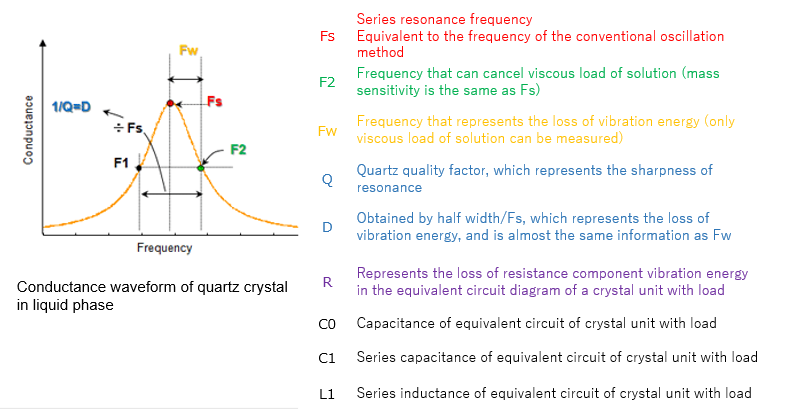In addition to the above frequencies, "Q" which indicates the ease of resonance of a quartz crystal and "D" which is the reciprocal of Q and shows the energy loss can be obtained.
The D can be calculated by the attenuation method as well as the admittance analysis method. The calculation method is different, but the values obtained from the calculations are the same.
The equivalent circuit parameters "R (R1)", "C0", "C1" and "L1" which shows the characteristics of the crystal resonator also can be obtained.
Like Fw and D, R is one of the parameters that represent the loss of vibrational energy.

## Analysis mode

We developed a method to calculate the viscoelastic coefficient and other physical properties from each frequency parameter of the fundamental and triple waves. As a result, a maximum of seven physical properties can be obtained. Discussions can be made, which could not be achieved by using only frequency, based on general physical properties.

Physical property Name overview
G' Storage modulus G '(G prime) is the component in which the energy of the strain of an object is stored as stress.It is one of the parameters that represents the elastic component of the viscoelastic body.
G" Loss modulus G "(G double prime) is a component that is lost when energy is converted to other energy such as heat and is a parameter that represents the viscosity component of viscoelasticity.
tan δ Loss coefficient It is calculated as G "/ G '.
A value of 0 means a solid (Viscosity component is zero), a value of ∞ means a solution (Elastic component is zero).Viscoelastic body shows intermediate value.
μ Elastic modulus A physical quantity that describes the difficulty of change. Also known as modulus of rigidity.In Pro measurement, it is one of the parameters that show the elastic component of the viscoelastic body.
η Viscosity A physical quantity that represents the characteristic of a substance to deform when a force is applied to the substance, and not to return to its original shape even after the force is removed. This is mainly liquid, but viscoelastic thin film analysis shows the viscosity component of deposits.
m Mass The mass of the adsorbate is calculated. If the adsorbate contains water, the mass includes the water.
h Film thickness The film thickness is calculated from the calculated mass and the density of the adsorbate.

The following is an actual example of a physical property evaluation of substances that adsorbed on the sensor.

### ex. 1)　Viscoelastic analysis of biotin DNA 60 mer.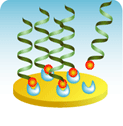For adsorbates with low moisture content such as hard film and hydrophobic film, viscoelastic analysis cannot be applied, but it is possible to calculate the mass (m) and film thickness (h) by using analysis modes such as Sauerbrey equation.In this example, the formation of SAM to the surface of the gold electrode is monitored. Fw, which represents the loss of vibrational energy, hardly changes, and only the adsorption amount of SAM is measured.Other measurement examples of such analysis are small globular proteins and polymer thin films.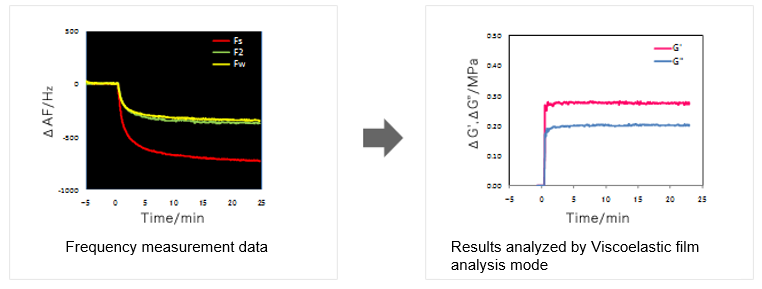### ex. 2)Physical property evaluation of self-assembled monolayer (SAM film)For adsorbates with low moisture content such as hard film and hydrophobic film, viscoelastic analysis cannot be applied, but it is possible to calculate the mass (m) and film thickness (h) by using analysis modes such as Sauerbrey equation.In this example, the formation of SAM to the surface of the gold electrode is monitored. Fw, which represents the loss of vibrational energy, hardly changes, and only the adsorption amount of SAM is measured.Other measurement examples of such analysis are small globular proteins and polymer thin films.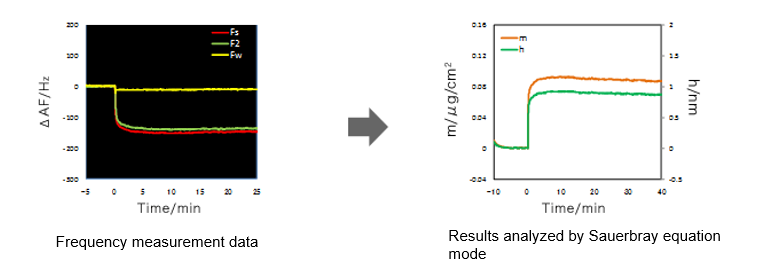### ex. 3)　Gelation measurement of agarose gel.

In addition to adsorbates, the viscoelastic coefficient of viscoelastic solutions, semi-solids, and solids that contact with the surface of the sensor are calculated.The sample volume that is used for measurement is 400 to 550μL. However, In the case of no agitation, the measurement can be performed from about 10μL (droplets on the electrode).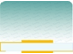• High-concentration protein solution.
• Colloid solution.
• Polymer solution.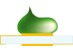• Grease and Oil.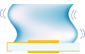• Gel.
• Gum.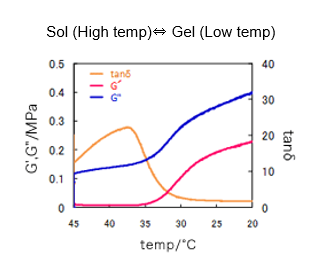Agarose gel changes from sol to gel when the temperature is lowered. This is a measurement example of the gelation process.

Gelation of agarose started as the elastic component G 'increased from around 36 ° C and the loss coefficient tanδ decreased.

### HOW TO

This website use cookies to obtain and use access data to understand the convenience and usage of customers. If you agree to use cookies, click "I Accept".